## B tree in c++

A B tree in c++ is a specialized multiway tree designed especially for use on disk. In a B-tree, each node may contain a large no. of keys. The no. of subtrees of each node, then, may also be large. A B tree in c++ is designed to branch out in this large number of directions and to contain a lot of keys in each node so that the height of the tree is relatively small. This means that only a small number of nodes must be read from disk to retrieve an item. The goal is to get fast access to the data, and with disk drives, this means reading a very small number of records. Remember that a large node size (with lots of keys in the node) also fits with the fact that with a disk drive one can usually read a fair amount of data at once.

### B Tree Example and Implementation in C++

The following is an example of a B-tree of order 5. This means that (other than the root node) all internal nodes have at least ceil(5 / 2) = ceil(2.5) = 3 children (and hence at least 2 keys). Of course, the maximum number of children that a node can have is 5 (so that 4 is the maximum number of keys). According to condition 4, each leaf node must contain at least 2 keys. In practice, B-trees usually have order a lot bigger than 5.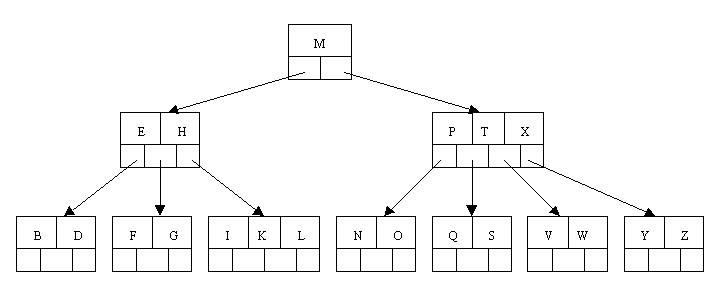### Inserting a New Item in B Tree

• Let’s work our way through an example similar to that given by Kruse. Insert the following letters into what is originally an empty B-tree of order 5: C N G A H E K Q M F W L T Z D P R X Y S Order 5 means that a node can have a maximum of 5 children and 4 keys. All nodes other than the root must have a minimum of 2 keys. The first 4 letters get inserted into the same node, resulting in this picture:• When we try to insert the H, we find no room in this node, so we split it into 2 nodes, moving the median item G up into a new root node. Note that in practice we just leave the A and C in the current node and place the H and N into a new node to the right of the old one.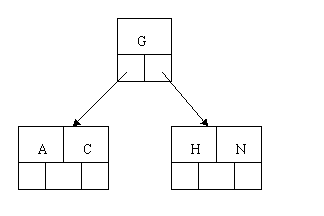• Inserting E, K, and Q proceeds without requiring any splits: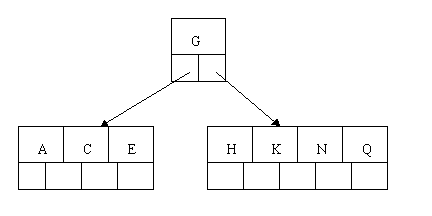• Inserting M requires a split. Note that M happens to be the median key and so is moved up into the parent node.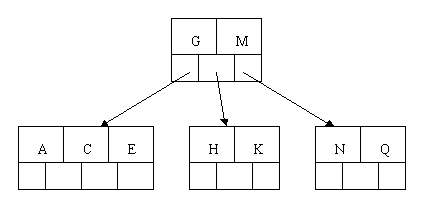• The letters F, W, L, and T are then added without needing any split.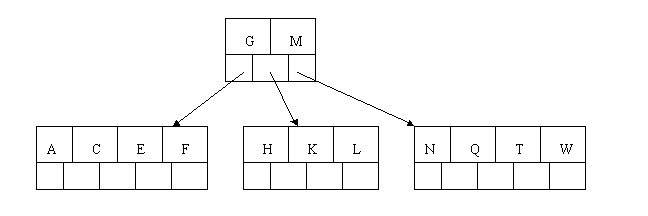• When Z is added, the rightmost leaf must be split. The median item T is moved up into the parent node. Note that by moving up the median key, the tree is kept fairly balanced, with 2 keys in each of the resulting nodes.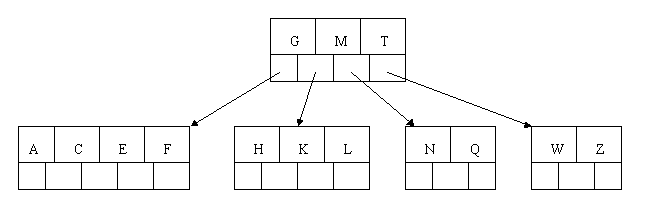• The insertion of D causes the leftmost leaf to be split. D happens to be the median key and so is the one moved up into the parent node. The letters P, R, X, and Y are then added without any need of splitting: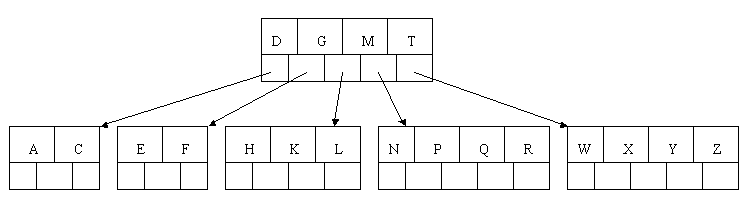• Finally, when S is added, the node with N, P, Q, and R split, sending the median Q up to the parent. However, the parent node is full, so it splits, sending the median M up to form a new root node. Note how the 3 pointers from the old parent node stay in the revised node that contains D and G.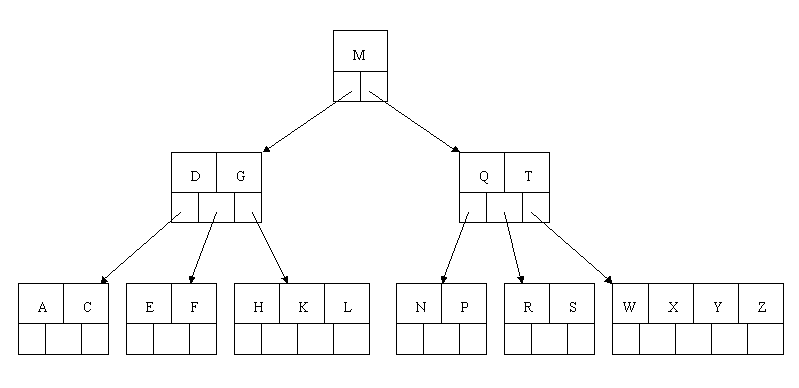### Deleting an Item in B Tree

• In the B-tree as we left it at the end of the last section, delete H. Of course, we first do a lookup to find H. Since H is in a leaf and the leaf has more than the minimum number of keys, this is easy. We move the K over where the H had been and the L over where the K had been. This gives: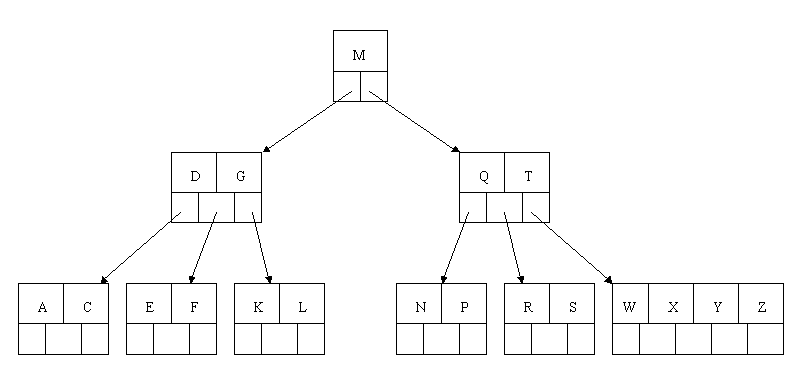• Next, delete the T. Since T is not in a leaf, we find its successor (the next item in ascending order), which happens to be W, and move W up to replace the T. That way, what we really have to do is to delete W from the leaf, which we already know how to do, since this leaf has extra keys. In ALL cases we reduce deletion to a deletion in a leaf, by using this method.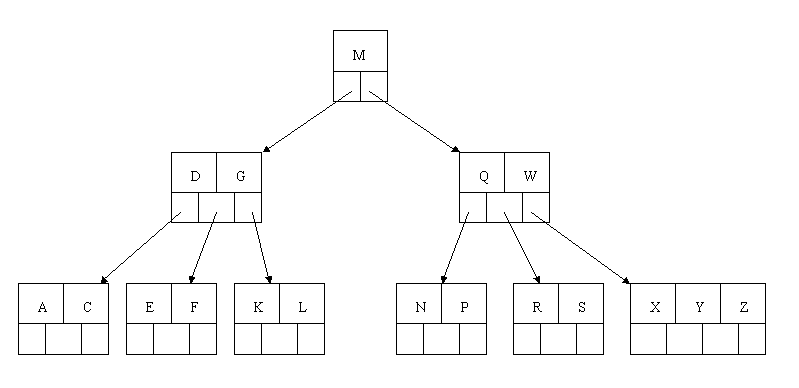• Next, delete R. Although R is in a leaf, this leaf does not have an extra key; the deletion results in a node with only one key, which is not acceptable for a B-tree of order 5. If the sibling node to the immediate left or right has an extra key, we can then borrow a key from the parent and move a key up from this sibling. In our specific case, the sibling to the right has an extra key. So, the successor W of S (the last key in the node where the deletion occurred), is moved down from the parent, and the X is moved up. (Of course, the S is moved over so that the W can be inserted in its proper place.)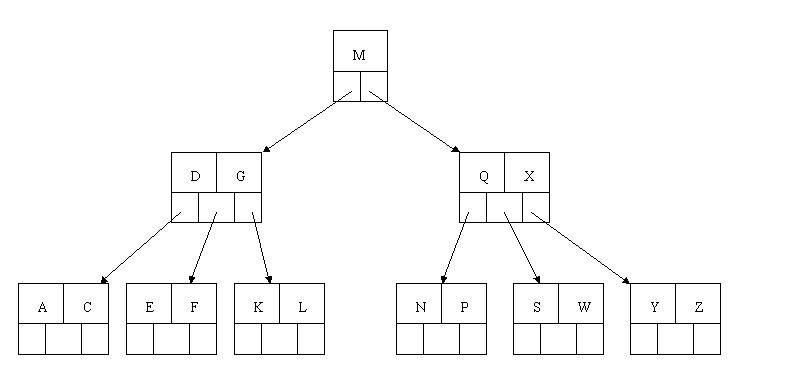• Finally, let’s delete E. This one causes lots of problems. Although E is in a leaf, the leaf has no extra keys, nor do the siblings to the immediate right or left. In such a case the leaf has to be combined with one of these two siblings. This includes moving down the parent’s key that was between those of these two leaves. In our example, let’s combine the leaf containing F with the leaf containing A C. We also move down the D.• Of course, you immediately see that the parent node now contains only one key, G. This is not acceptable. If this problem node had a sibling to its immediate left or right that had a spare key, then we would again “borrow” a key. Suppose for the moment that the right sibling (the node with Q X) had one more key in it somewhere to the right of Q. We would then move M down to the node with too few keys and move the Q up where the M had been. However, the old left subtree of Q would then have to become the right subtree of M. In other words, the N P node would be attached via the pointer field to the right of M’s new location.
• Since in our example we have no way to borrow a key from a sibling, we must again combine with the sibling, and move down the M from the parent. In this case, the tree shrinks in height by one.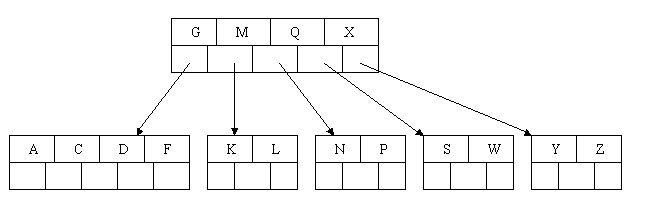### B tree Example Program:

```
#include
#include
#include
const int MAX = 4 ;
const int MIN = 2 ;
struct btnode
{
int count ;
int value[MAX + 1] ;
btnode *child[MAX + 1] ;
} ;
class btree
{
private :
btnode *root ;
public :
btree( ) ;
void insert ( int val ) ;
int setval ( int val, btnode *n, int *p, btnode **c ) ;
static btnode * search ( int val, btnode *root, int *pos ) ;
static int searchnode ( int val, btnode *n, int *pos ) ;
void fillnode ( int val, btnode *c, btnode *n, int k ) ;
void split ( int val, btnode *c, btnode *n,
int k, int *y, btnode **newnode ) ;
void del ( int val ) ;
int delhelp ( int val, btnode *root ) ;
void clear ( btnode *root, int k ) ;
void copysucc ( btnode *root, int i ) ;
void restore ( btnode *root, int i ) ;
void rightshift ( int k ) ;
void leftshift ( int k ) ;
void merge ( int k ) ;
void show( ) ;
static void display ( btnode *root ) ;
static void deltree ( btnode *root ) ;
~btree( ) ;
} ;

btree :: btree( )
{
root = NULL ;
}
void btree :: insert ( int val )
{
int i ;
btnode *c, *n ;
int flag ;
flag = setval ( val, root, &i, &c ) ;
if ( flag )
{
n = new btnode ;
n -> count = 1 ;
n -> value = i ;
n -> child = root ;
n -> child = c ;
root = n ;
}
}
int btree :: setval ( int val, btnode *n, int *p, btnode **c )
{
int k ;
if ( n == NULL )
{
*p = val ;
*c = NULL ;
return 1 ;
}
else
{
if ( searchnode ( val, n, &k ) )
cout << endl << "Key value already exists." << endl ;
if ( setval ( val, n -> child[k], p, c ) )
{
if ( n -> count < MAX )
{
fillnode ( *p, *c, n, k ) ;
return 0 ;
}
else
{
split ( *p, *c, n, k, p, c ) ;
return 1 ;
}
}
return 0 ;
}
}
btnode * btree :: search ( int val, btnode *root, int *pos )
{
if ( root == NULL )
return NULL ;
else
{
if ( searchnode ( val, root, pos ) )
return root ;
else
return search ( val, root -> child[*pos], pos ) ;
}
}
int btree :: searchnode ( int val, btnode *n, int *pos )
{
if ( val < n -> value )
{
*pos = 0 ;
return 0 ;
}
else
{
*pos = n -> count ;
while ( ( val < n -> value[*pos] ) && *pos > 1 )
( *pos )-- ;
if ( val == n -> value[*pos] )
return 1 ;
else
return 0 ;
}
}
void btree :: fillnode ( int val, btnode *c, btnode *n, int k )
{
int i ;
for ( i = n -> count ; i > k ; i-- )
{
n -> value[i + 1] = n -> value[i] ;
n -> child[i + 1] = n -> child[i] ;
}
n -> value[k + 1] = val ;
n -> child[k + 1] = c ;
n -> count++ ;
}
void btree :: split ( int val, btnode *c, btnode *n,
int k, int *y, btnode **newnode )
{
int i, mid ;

if ( k <= MIN )
mid = MIN ;
else
mid = MIN + 1 ;

*newnode = new btnode ;

for ( i = mid + 1 ; i <= MAX ; i++ )
{
( *newnode ) -> value[i - mid] = n -> value[i] ;
( *newnode ) -> child[i - mid] = n -> child[i] ;
}

( *newnode ) -> count = MAX - mid ;
n -> count = mid ;

if ( k <= MIN )
fillnode ( val, c, n, k ) ;
else
fillnode ( val, c, *newnode, k - mid ) ;

*y = n -> value[n -> count] ;
( *newnode ) -> child = n -> child[n -> count] ;
n -> count-- ;
}
void btree :: del ( int val )
{
btnode * temp ;

if ( ! delhelp ( val, root ) )
cout << endl << "Value " << val << " not found." ;
else
{
if ( root -> count == 0 )
{
temp = root ;
root = root -> child ;
delete temp ;
}
}
}
int btree :: delhelp ( int val, btnode *root )
{
int i ;
int flag ;

if ( root == NULL )
return 0 ;
else
{
flag = searchnode ( val, root, &i ) ;
if ( flag )
{
if ( root -> child[i - 1] )
{
copysucc ( root, i ) ;
flag = delhelp ( root -> value[i], root -> child[i] ) ;
if ( !flag )
cout << endl << "Value " << val << " not found." ;
}
else
clear ( root, i ) ;
}
else
flag = delhelp ( val, root -> child[i] ) ;
if ( root -> child[i] != NULL )
{
if ( root -> child[i] -> count < MIN )
restore ( root, i ) ;
}
return flag ;
}
}
void btree :: clear ( btnode *root, int k )
{
int i ;
for ( i = k + 1 ; i <= root -> count ; i++ )
{
root -> value[i - 1] = root -> value[i] ;
root -> child[i - 1] = root -> child[i] ;
}
root -> count-- ;
}
void btree :: copysucc ( btnode *root, int i )
{
btnode *temp = root -> child[i] ;

while ( temp -> child )
temp = temp -> child ;

root -> value[i] = temp -> value ;
}
void btree :: restore ( btnode *root, int i )
{
if ( i == 0 )
{
if ( root -> child  -> count > MIN )
leftshift ( 1 ) ;
else
merge ( 1 ) ;
}
else
{
if ( i == root -> count )
{
if ( root -> child[i - 1] -> count > MIN )
rightshift ( i ) ;
else
merge ( i ) ;
}
else
{
if ( root -> child[i - 1] -> count > MIN )
rightshift ( i ) ;
else
{
if ( root -> child[i + 1] -> count > MIN )
leftshift ( i + 1 ) ;
else
merge ( i ) ;
}
}
}
}
void btree :: rightshift ( int k )
{
int i ;
btnode *temp ;

temp = root -> child[k] ;

for ( i = temp -> count ; i > 0 ; i-- )
{
temp -> value[i + 1] = temp -> value[i] ;
temp -> child[i + 1] = temp -> child[i] ;
}

temp -> child = temp -> child ;
temp -> count++ ;
temp -> value = root -> value[k] ;
temp = root -> child[k - 1] ;
root -> value[k] = temp -> value[temp -> count] ;
root -> child[k] -> child  = temp -> child[temp -> count] ;
temp -> count-- ;
}
void btree :: leftshift ( int k )
{
btnode *temp ;

temp = root -> child[k - 1] ;
temp -> count++ ;
temp -> value[temp -> count] = root -> value[k] ;
temp -> child[temp -> count] = root -> child[k] -> child ;
temp = root -> child[k] ;
root -> value[k] = temp -> value ;
temp -> child = temp -> child ;
temp -> count-- ;
for ( int i = 1 ; i <= temp -> count ; i++ )
{
temp -> value[i] = temp -> value[i + 1] ;
temp -> child[i] = temp -> child[i + 1] ;
}
}
void btree :: merge ( int k )
{
btnode *temp1, *temp2 ;
temp1 = root -> child[k] ;
temp2 = root -> child[k - 1] ;
temp2 -> count++ ;
temp2 -> value[temp2 -> count] = root -> value[k] ;
temp2 -> child[temp2 -> count] = root -> child ;
for ( int i = 1 ; i <= temp1 -> count ; i++ )
{
temp2 -> count++ ;
temp2 -> value[temp2 -> count] = temp1 -> value[i] ;
temp2 -> child[temp2 -> count] = temp1 -> child[i] ;
}
for ( i = k ; i < root -> count ; i++ )
{
root -> value[i] = root -> value[i + 1] ;
root -> child[i] = root -> child[i + 1] ;
}
root -> count-- ;
delete temp1 ;
}
void btree :: show( )
{
display ( root ) ;
}
void btree :: display ( btnode *root )
{
if ( root != NULL )
{
for ( int i = 0 ; i < root -> count ; i++ )
{
display ( root -> child[i] ) ;
cout << root -> value[i + 1] << "\t" ;
}
display ( root -> child[i] ) ;
}
}
void btree :: deltree ( btnode *root )
{
if ( root != NULL )
{
for ( int i = 0 ; i < root -> count ; i++ )
{
deltree ( root -> child[i] ) ;
delete ( root -> child[i] ) ;
}
deltree ( root -> child[i] ) ;
delete ( root -> child[i] ) ;
}
}

btree :: ~btree( )
{
deltree ( root ) ;
}

void main( )
{
btree b ;
int arr[ ] = { 27, 42, 22, 47, 32, 2, 51, 40, 13 } ;
int sz = sizeof ( arr ) / sizeof ( int ) ;
for ( int i = 0 ; i < sz ; i++ )
b.insert ( arr[i] ) ;
cout << "B-tree of order 5:" << endl ;
b.show( ) ;
b.del ( 22 ) ;
b.del ( 11 ) ;
cout << "\n\nB-tree after deletion of values:" << endl ;
b.show( ) ;
}

```